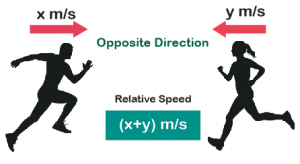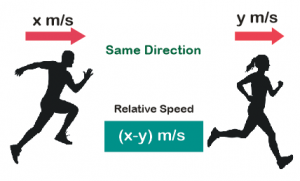# Relative Speed Concept and Questions for CAT Exam

Relative Speed Concept and Questions for CAT Exam
5 (100%) 19 vote[s]

Relative speed concept is can be of great help in solving many questions from time speed and distance in CAT exam. The concept is particularly critical for problems based on trains, and meeting points The idea is simple to understand, and we mainly need to know the working of the concept in the following cases

• Two bodies moving in the same direction
• Two bodies moving in the opposite direction

Let us try to understand it by taking a practical example. Consider a person A standing on a platform waiting for a train. In the meantime, a different train passes the same platform and takes t minutes to cross A.

If A tries to run towards this train such that both of them keep on moving in opposite directions, then the train will take a time tless which is less than t min to cross this person A.

On the other hand, if the person runs in the same direction in which the train is moving, then the train will require a time tmore, which is more than t min.Thus, the basic concept of relative speed is that, when two objects or bodies move in the same direction, the relative speed of one body with respect to the other is the difference between the speeds of the two. And when the two bodies are moving in the opposite direction, the relative speed of one body with respect to the other is the sum of the speeds of the two.

## Relative Speed Formulas

If two objects are moving with speeds X  and Y, their relative speed is

• X + Y, if they are moving in the opposite direction
• XY, if they are moving in the same direction.## Solved Problems on Relative Speed

Question 1: The distance between two cities A and B is 80 km. A motorcycle rider starts from A towards B at 7 am at a speed of 10 km/hr. Another motorcyclist starts from B towards A at 8 am at a speed of 25 km/hr. At what time will they cross each other?

Explanation:

In one hour, the earlier rider covers a distance of 10 km.

The distance between the two = 80 – 10 = 70 km.

Hence, the two riders approach each other with a relative speed of 10 + 25 = 35 kmph.

The time taken = 70/35 = 2 hrs. Therefore, they meet each other at (8 + 2) = 10 am.

Question 2: Two trains start at the same time from two stations and proceed towards each other at the rate of 20 km/hr and 25 km/hr respectively. When they meet, it is found that one train has traveled 80 km more than the other. Find the distance between the two stations.

Explanation:

In one hour, one train travels 5 km more than the other. The trains meet in 80/5 = 16 hours.

Therefore, the distance = (20 + 25) x 16 = 720 km.

Question 3:  Two trains start at the same time from Aligarh and Delhi and proceed towards each other at the rate of 16 km/hr and 21 km/hr respectively. When they meet, it is found that one train has traveled 60 km more than the other. The distance between the two stations is

Explanation:

Both the trains are coming closer to each other by (21 – 16), i.e., 5 km every hour.

They would meet after 60/5 = 12 hrs. Hence, the distance between the 2 stations = (21 + 16) x 12 = 37 x 12 = 444 km.

Question 4: A starts from X at 9:00 am and reaches Y at 1:00 pm. B starts from Y at 9:00 am and reaches X at 3 pm. At what time do the two meet?

Explanation:

Assuming the distance between X and Y to be d, since A and B take 4 hours and 6 hours respectively to cover the distance, their speeds are $\frac{d}{4}\;and\;\frac{d}{6}$ respectively.

Thus the time taken to meet will be $\frac{d}{{\frac{d}{4} + \frac{d}{6}}} = \frac{1}{{\frac{1}{4} + \frac{1}{6}}} = \frac{{4 \times 6}}{{4 + 6}} = 2.4\;hrs$ after 9 am, i.e., 11:24 am.

Alternate approach:

The above question can also be solved by assuming the distance to be the lcm of the time taken by them.

i.e. distance = LCM (4, 6) = 12 km.

So, their speeds will be 12/4=3 kmph and 12/6 = 2 kmph respectively.

Therefore, their relative speed = 3+2 = 5 kmph.

Hence, the time taken by them to meet = 12/5 = 2.4 hrs

Question 5: Two boats, traveling at 5 and 10 km per hour, head directly towards each other. They begin at a distance of 20 km from each other. How far apart are they (in km) one minute before they collide? [CAT 2004]

(1) 1/12

(2) 1/6

(3) 1/4

(4) 1/3

Explanation:

Relative speed of two boats =5+10=15 km/hr i.e. in 60 min they cover (together) = 15 km

In 1 min they will cover (together) =$\frac{{15}}{{60}} = \frac{1}{4}km$

Question 6: A train X departs from station A at 11.00 a.m. for station B, which is 180 km away. Another train Y departs from station B at 11.00 a.m. for station A. Train X travels at an average speed of 70 kms/hr and does not stop anywhere until it arrives at station B. Train Y travels at an average speed of 50 kms/hr, but has to stop for 15 minutes at station C, which is 60 kms away from station B enroute to station A. Ignoring the lengths of the trains, what is the distance, to the nearest km, from station A to the point where the trains cross each other? [CAT 2001]
(1) 112

(2) 118

(3) 120

(4) None of these

Explanation:

Time taken by train Y to reach station C which is 60 km away = $\frac{{60}}{{50}} = 1\frac{1}{5}$ hour = 1 hr 12 min
Train Y then stops in station C for 15 minutes.
Time taken by train Y to leave station C = 1 hr 12 min + 15 minutes = 1 hr 27 min

Distance travelled by train X in this 1 hr 27 minutes = $70 \times 1\frac{{27}}{{60}} = 70 \times \frac{{87}}{{60}}$ = 101.5 km

After 1 hr 27 minutes, distance between train X and Y = 180 – 60 – 101.5 = 18.5 km
Relative speed = 70 + 50 = 120 km/hr

Time taken by the trains to meet each other = $\frac{{18.5}}{{120}} = \frac{{37}}{{240}}$ hr

Distance travelled by train X in this time = $70 \times \frac{{37}}{{240}}$ = 10.8 km

Required distance from station A to the point where trains cross each other = 101.5 + 10.8 = 112.3 ≈ 112 km

Question 7: Navjivan Express from Ahmedabad to Chennai leaves Ahmedabad at 6:30 am and travels at 50 km per hour towards Baroda situated 100 km away. At 7 : 00 am Howrah-Ahmedabad Express leaves Baroda towards Ahmedabad and travels at 40 km per hour. At 7 : 30 Mr. Shah, the traffic controller at Baroda realises that both the trains are running on the same track. How much time does he have to avert a head-on collision between the two trains? [CAT 1999]

(1) 15 minutes

(2) 20 minutes

(3) 25 minutes

(4) 30 minutes

Explanation:

The distance between Ahmedabad and Baroda given is 100 Km

Navjivan express starts at 6:30 am at 50 Km/hr and Howrah expresses starts at 7:00 am at 40 Km/hr.

Therefore, the distance covered by Navjivan express in 30 minutes (by 7 am) is 25 Km/hr.

Hence, at 7 am, the distance between the two trains is 75 Kms and they are travelling in opposite direction with a relative speed of 50+40=90 Km/hr.

So, the time taken them to meet is $\frac{75}{90}\times60=50 minutes$.

Also, the problem was realized after thirty minutes. Therefore, the time left to avoid collision is 50-30 = 20 minutes

## Time Speed and Distance